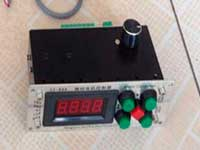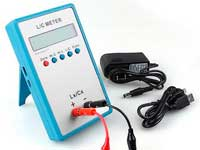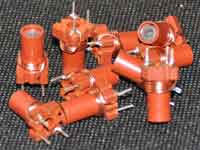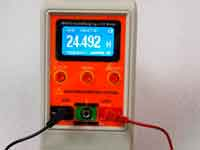The self-resonance frequency - 4.4 out of 5 based on 5 votes
Rating 4.40 (5 Votes)

# The self-resonance frequencyIn the early days of the Age of Radio, it was discovered that the helix inductor is not an ideal inductor. At a certain frequency it is in the resonance mode even in the absence of external capacitance, and above this frequency the impedance of the coil is capacitive. To explain this phenomenon assumed that in addition to self-inductance real coil has a self-capacitance (conceivably between adjacent turns) and the coil is presented as a model of lumped RLC elements, where L — inductance, C — self-capacitance, and R takes into account the various losses in the coil. Such a model has only one resonant frequency, called the self-resonance frequency of the coil (SRF). This model became a classical model of the real coil in all textbooks.

Because the coil, in the majority of practical applications, is working at frequencies far below the SRF, the purpose of the designer is the providing this condition. The majority of engineers with this purpose tried to reduce this "inter-turn stray capacitance". In case, if the coil operates at frequencies close to SRF, as for example in a helical resonator or Tesla coils, RLC-model gives incorrect results, but in such cases, had developed an alternative calculation algorithms and all designers were satisfied and not really turned over in one's mind about the reasons for such inconsistencies.

In Digital Age appeared applications that gave the opportunity to simulate any high-frequency devices with the high degree of accuracy, the so-called electromagnetic simulators. There are powerful packages such as CST Studio, HFSS, and many others. Let's test the single-layer helical coil in the HFSS. In the first model, place the coil over a perfectly conducting surface and excite it by a lumped port with an internal resistance of 50 MOhm. The second end of the coil is grounded. The simulation will be done in the mode of "HFSS Design" using finite element method.A second coil we simulate by the "Design HFSS-IE" method using the method of moments. We excite the coil through the short leads from the same lumped port. Since the coil isn't grounded in this model, the first resonance is half-wave resonance.The study we have received the graphs of the impedance at the terminals of the source depending on frequency. The graphs show that the helical coil has many resonances. The conclusion from this is that the coil isn't a single LC-circuit with its self-inductance and stray self-capacitance as lumped elements, but it's transmission line with distributed parameters. The fact that the transmission line consists of a single wire does not have to be confusing. You know, that resonance phenomenon is observed even in one wire, it shows an example of a dipole. The existence of wave phenomena in wires displays the fact that electromagnetic interaction propagates with a finite speed. And coil in this respect isn't far from the dipole, because, despite its small size, the length of the wire which it is wound, have dimensions comparable to the wavelength. The self-resonant frequency of the dipole one can quite easily determine, by knowing its length. For a coil, it's not so easy.

In electrodynamics textbooks, you can find a description of the operation of the spiral waveguides with surface electromagnetic (EM) waves propagating along the helical wire. Such waveguides are used as slow-wave structures in spiral antennas and traveling wave tubes. In such waveguides, the length of one turn and the winding pitch are comparable with the wavelength.The phase velocity of the wave along the axis of the helical waveguide is much lower than the speed of light, and its application as a slow-wave structure is based on this.The relative phase velocity of the wave along the axis of such a waveguide depends only on the geometry of the helix and is not dependent on frequency since the EM-wave almost propagates only along the wire of the helix.

In the coil, the length of each turn, the length of the winding, and the pitch of winding are much smaller than the wavelength. In this case, besides the main mode along the wire, there exist higher modes directly along the axis of the helix. In other words, EM wave propagates not only along the length of the wire but part of her "jumps from turn to turn". The relative phase velocity along the axis of the coil is determined by the following approximate expression:where:

• λ0 - wavelength of the operating frequency in free space
• D, p - diameter and pitch of the winding
• c - velocity of the light

As can be seen from the formula, the speed depends on the coil diameter, winding pitch and the wavelength. In fact, the coil is the same helical waveguide with slow waves, but it's working in a different mode.
Due to the presence of high-order modes of the electromagnetic wave, the frequency of the first resonance of the coil is always higher than the frequency calculated from a length of wire. For this reason, higher frequency resonances are not multiples to the first and to each other.
The short coils have the subsequent resonances which are separated from the first far higher in frequency, that you can see in the results of HFSS simulation:At frequencies much below the first resonance frequency the spatial delay much less than the period of oscillations of the EM field. The field around the coil is a static solenoid field and the wave propagation speed along its axis can be ignored. In this case, the lumped RLC model will be fully working and accurately displays the behavior of the coil. One has only to remember that the stray self-capacitance is not a static capacity between the turns. The coils of all three models are working in this mode at the SW range and below. However, the frequency of the first resonance begins to show the wave effects associated with a limited speed of transmission of electromagnetic coupling and the coil should only be considered as a helical waveguide. In this case, the RLC model is not right. Moreover, it leads to a wrong understanding of the resonance phenomena mechanism in the coil.
The coil as a set of lumped RLC elements and the coil as a circuit with distributed parameters are the two different models of the real coil. The first model is based on the assumption that the current density in all turns is the same, so it is limited and applicable only at low frequencies, the second is complete and applicable at any frequency.

I would especially like to note the following circumstance. At low frequencies, where, as we have seen, the RLC model is valid, we can assume that the inductance and self-capacitance of the coil don't depend on frequency and are determined only by the geometry of the winding. It is a well-known fact which is fixed for example in the Nagaoka's formula. But in reality, the parameters of a helical transmission line depends on the frequency. Not just vax, but capacity and inductance per unit length. Only at low frequencies, this dependence is negligible, however, at frequencies close to the first resonance the values of inductance and self-capacitance of the coil begin to visibly "float" by frequency. In the end, we are faced with a situation that these values, measured or calculated at a low frequency, not suitable for calculating the self-resonant frequency according to the Thomson's formula. The calculation will give an incorrect result! Therefore, to calculate the resonant frequency of the coil is necessary to use a more complex numerical method from . The second, any object near the coil, including measurement probe, influences on transmission line parameters. In this case, the self-resonance frequency is moved from its actual value for "free" coil.

If the designer needs to create a coil with the minimum dimensions and having the maximum self-resonant frequency, then the best winding will be with the distance between the turns equal to the wire diameter, and with the ratio l/D ≈ 1..1,5. In practice, should to stick to the following simple rules: if the total length of the coil wire is less than a quarter wavelength at the highest operating frequency, the coil will operate below their first resonance.

References:

Сomments from anonymous guests are enabled with moderation.

#### Coil Winding Machine###### US \$187.53
The system consists of individual components including a controller, a stepping motor, a foot switch, power adapter and output shaft. This design makes it very easy for you to integrate the system into your own project.

#### LC Meter LC200A###### US \$37.99
Measuring Capacitance Range:
0.01 pF - 100mF
Measuring Inductance Range:
0.001 uH - 100H
Measurement accuracy:1%.

#### RF Inductors###### US \$6.43
Variable Ferrite Core Inductor coil set 3.5Turns 25-100MHZ

#### Auto Ranging LCR Meter###### US \$63.07
Meter can measure 0.00pF to 100.00mF capacitance, 0.000uH to 100.00H inductance and 0.00? to 10.000M? resistance. Very suitable for small value capacitor and inductor measurement.